<< Previous    1...   50  51    53  54  ...56    Next >>

#52 The outer and inner Tree of Life basis of E8 and E8×E8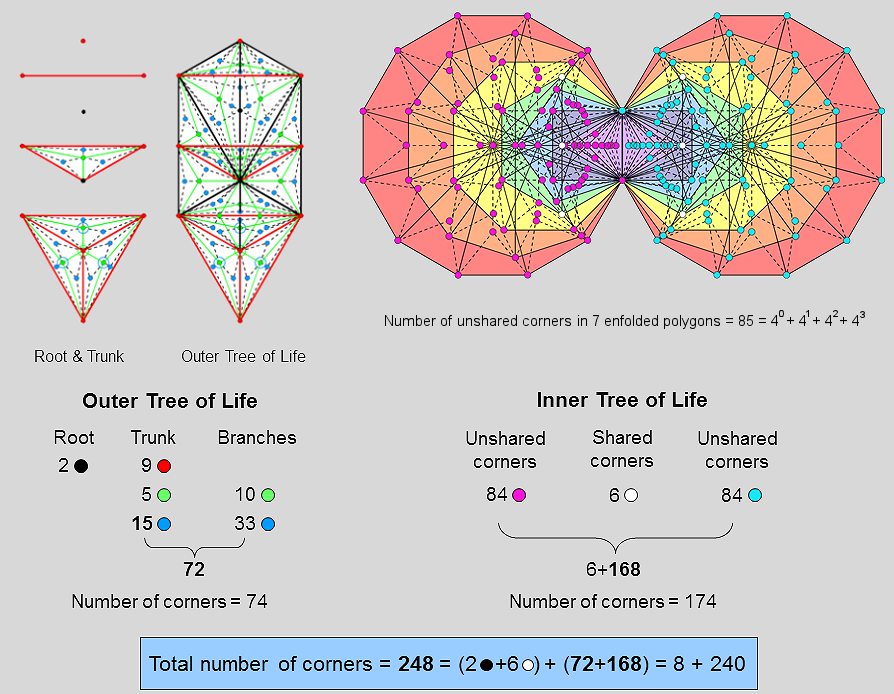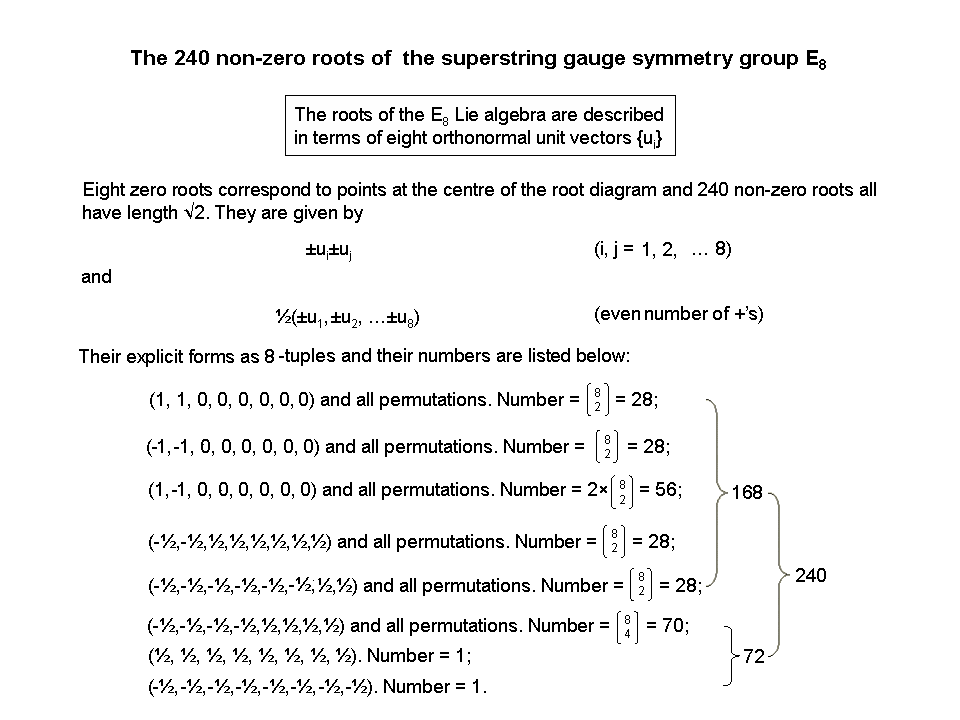240 8-tuples represent the 240 non-zero roots of E8 as 8-dimensional vectors of length √2. Their counterparts in the separate outer & inner Trees of Life with Type B polygons are the 240 corners of their 426 triangles that are neither in the root of the outer Tree of Life nor shared with the inner Tree of Life. The 8 corners that are either in it or shared correspond to the 8 zero roots of E8.

The outer & inner Tree of Life basis of the heterotic superstring symmetry group E8

Outer Tree of Life
A Type B triangle is composed of 9 triangles with 3 external corners and 4 corners that are inside it. The 16 Type B triangles of the outer Tree of Life have 10 corners on their 22 sides and (16×4=64) internal corners of their (16×9=144) triangles with (16×12=192) sides. The outer Tree of Life comprises its root, its trunk and its branches. In terms of the 74 corners of its 144 triangles, the root consists of two corners whose projection onto the plane containing the (7+7) enfolded polygons of the inner Tree of Life are the two endpoints of their shared root edge. One corner is located at Daath, which is the centre of the triangle formed by Chokmah, Binah & Tiphareth. The other corner coincides with Tiphareth, which is the centre of the Tree of Life in both a geometrical and a metaphysical sense. It is where the root joins the trunk of the Tree of Life, which is defined as the sequence:

point-line-triangle-tetrahedron

symbolising the integers 1, 2, 3 & 4, Tiphareth being located at the lowest corner of the triangle in this sequence. The corners making up the trunk are composed of the 9 red corners of all 16 primary triangles, the 5 green centres of its 5 primary triangles and the (5×3=15) blue corners of their (5×9=45) internal triangles. The branches are the remainder of the outer Tree of Life outside the root & trunk. They comprise 11 primary triangles with 10 green centres and (3×11=33) blue corners.

The 74 corners comprise the two black corners forming the root and the 72 corners of the trunk (29 corners) and branches (43 corners). The 16 primary triangles have (10+16=26) corners & centres, whereandso that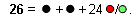This compares with the 26 dimensions predicted by quantum mechanics for spinless strings being made up of the time dimension, the longitudinal dimension and 24 transverse dimensions comprising 9 dimensions of the 11-dimensional space-time predicted by M-theory and the 15 additional, bosonic string dimensions. The root of the outer Tree of Life is analogous to the time and longitudinal dimensions of such a string in 26-dimensional space-time. Neither of these participates in creating shape. Rather, in each case they are its root-like source. It means that the 9 dimensions are the trunk of space-time and the 15 bosonic string dimensions are its branches.

Inner Tree of Life
The 7 enfolded, Type B polygons have 47 sectors with 41 corners. Their (47×3=141) triangles have (41+47=88) corners (86 outside the root edge). The (7+7) enfolded, Type B polygons have (2×141=282) triangles with (2 + 2×86 = 174) corners. 282 is the number value of Aralim, the Order of Angels assigned to Binah. The centre, top and bottom of each hexagon coincide with corners of triangles belonging to the outer Tree of Life. These six white corners are shared and (174−6=168) corners are unshared. (168/2=84) corners (turquoise or pink) are associated with each set of 7 enfolded polygons, which have 85 unshared corners, where
84 = 12 + 32 + 52 + 72
and
85 = 40 + 41 + 42 + 43.
The (47×2=94) sectors of the (7+7) enfolded polygons have 80 corners. 87 corners of (47×3=141) triangles are associated with each set of 7 enfolded polygons when they are Type B. 80 is the number value of Yesod and 87 is the number value of Levanah, its Mundane Chakra. The separate outer and inner Trees of Life have (74+174=248) corners of (144+282=426) triangles. Excluding the root, there are 246 corners, where 246 is the number value of Gabriel, the Archangel of Yesod. 248 is the dimension of the rank-8, exceptional Lie group E8 appearing in E8×E8′ heterotic superstring theory. The 8 white or black corners symbolise the 8 simple roots of E8; the (72+168=240) other corners symbolise its 240 roots. The 72 corners in the trunk and branches symbolise the 72 roots of E6, the rank-6, exceptional Lie group that is a subgroup of E8. The 168 unshared corners in the inner Tree of Life denote the 168 roots of E8 that do not belong to E6. The 84:84 division of the superstring structural parameter 168 is characteristic of sacred geometries (see Article 64).

The (282+144=426) triangles in the combined outer & inner Trees of Life have 240 corners other than those forming the root. Alternatively, they have 240 corners other than the two endpoints of the root edge. This embodiment of the number 240 is characteristic of sacred geometries (see #1) because it quantifies the 240 roots of E8, which this website demonstrates is part of the divine paradigm.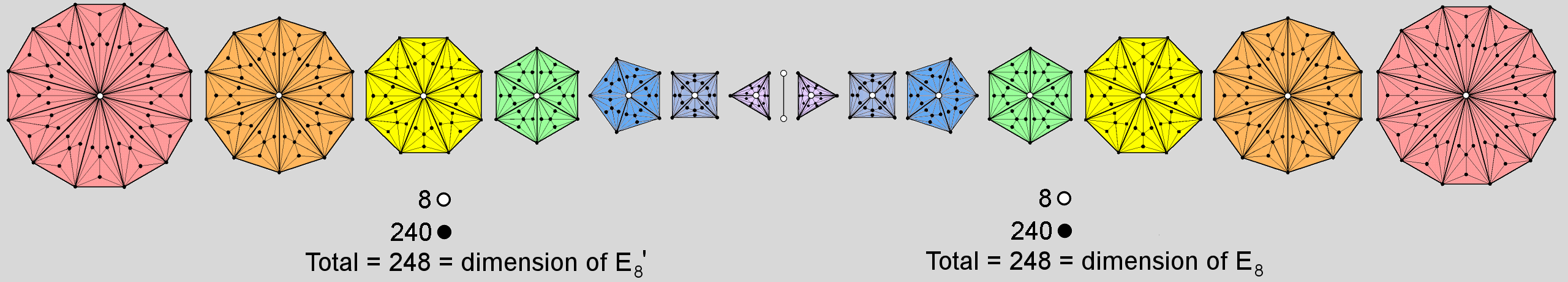The inner Tree of Life basis of the heterotic superstring symmetry group E8×E8

Each of the n sectors of a Type C n-gon is divided into three smaller sectors that are further divided into three triangles. The 9n triangles have (5n+1) corners. The 7 separate Type C polygons with 48 corners have 247 corners. Consider the two sets of 7 such polygons separated by the root edge. The two endpoints of the latter can be regarded as two corners of a triangle in the limit that its third corner collapses into its base. 248 corners are associated with each set of 7 polygons separated by the root edge. The first 6 separate polygons with 36 corners have 324 triangles with 186 corners. Hence, 187 corners are associated with the root edge and each set of the first 6 polygons. 248 is the number value of Raziel, the Archangel of Chokmah, and 187 is the number value of Auphanim, the Order of Angels assigned to this Sephirah.

The 248 corners associated with each set of 7 polygons and the separate root edge correspond to the 248 roots of the rank-8, exceptional Lie group E8. Their 7 centres and an endpoint of the root edge correspond to its 8 simple roots; the 240 other corners correspond to its 240 roots. The direct product E8×E8′ (one of the two possible superstring symmetry groups) is the consequence of the inner form of the Tree of Life having two halves, one of which is the mirror image of the other. The (7+7) Type C polygons and the root edge have 496 corners. 496 is the number value of Malkuth (material universe) and the dimension that a superstring symmetry group must have to make all its quantum anomalies disappear.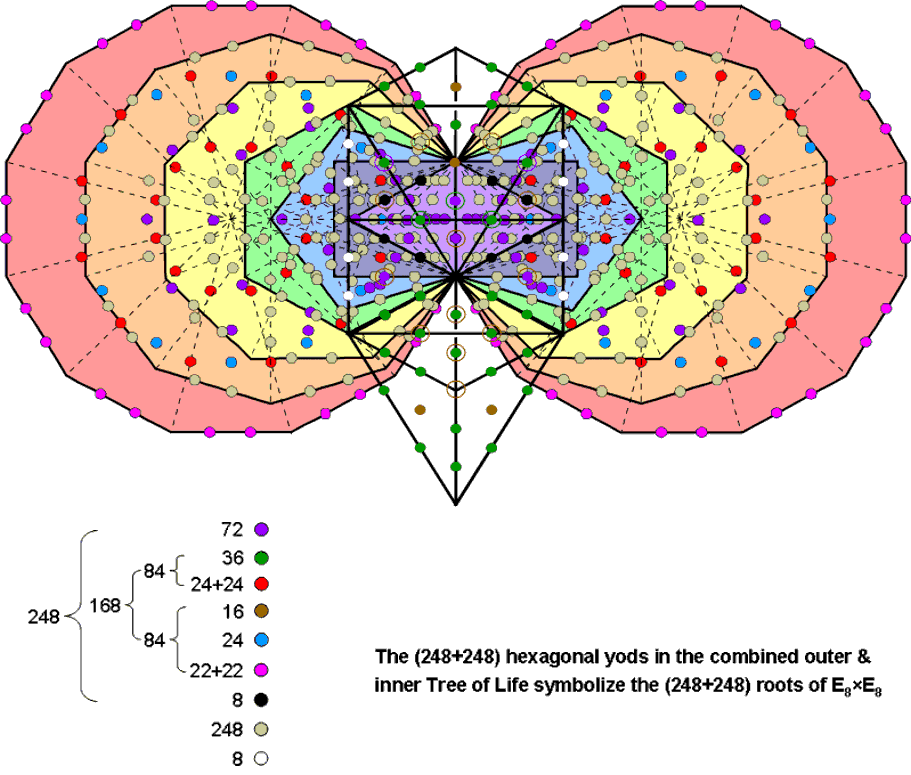The outer and inner Trees of Life embody E8×E8 heterotic superstring theory

The outer & inner Tree of Life basis of E8×E8

Outer Tree of Life
Turned into tetractyses, the 16 triangles contain 60 hexagonal yods consisting of the 16 brown hexagonal yods at their centres and the 44 hexagonal yods on their 22 sides (see diagram opposite). As four of these sides are sides of the sectors of the two hexagons belonging to the inner Tree of Life, the outer Tree of Life has (22−4=18) sides with (18×2= 36) green hexagonal yods that are unshared with its inner form as hexagonal yods, as well as (4×2=8) white hexagonal yods that are shared. Hence, the outer Tree has (16+36=52) unshared hexagonal yods. 52 is the 26th even integer, showing how YAHWEH with number value 26 determines the population of hexagonal yods that are intrinsic to the outer Tree. The two hexagonal yods on the Chesed-Geburah Path are the centres of the two violet triangles belonging to the (7+7) enfolded polygons, so that they are corners of tetractyses belonging to the latter. This means that 50 of the 52 hexagonal yods intrinsic to the outer Tree are hexagonal yods from the perspective of both Trees, showing how ELOHIM, the Godname of Binah with number value 50, prescribes this property.

Inner Tree of Life
Turned into tetractyses, the (47+47=94) sectors of the (7+7) enfolded polygons have 524 yods. They consist of 80 corners of 94 tetractyses and 444 hexagonal yods, where 80 is the number of Yesod. The latter comprise 94 hexagonal yods at the centres of tetractyses and (444−94=350) hexagonal yods that line their sides. Each dodecagon has 48 hexagonal yods lining sides of tetractyses. The 46 such yods outside the root edge consist of 22 pink hexagonal yods on its sides and 24 red hexagonal yods on internal sides of its 12 tetractyses. Hence, 46 = 22 + 24 and 350 = 46 + 2 + 46 + 256, where "2" denotes the two purple hexagonal yods on the root edge, there being 256 (=44) hexagonal yods outside it that line tetractyses in the first (6+6) enfolded polygons. Each set of the first six enfolded polygons has 35 sectors; the dodecagon has 12 sectors. (35+35=70) of the 94 hexagonal yods at centres of tetractyses belong to the first (6+6) enfolded polygons; they are coloured purple. The 24 remaining hexagonal yods belong to the two dodecagons; they are coloured blue. Therefore,

444 = 350 + 94

= 46 + 2 + 46 + 256 + 70 + 24

= 22 + 24 + 2 + 22 + 24 + 256 + 70 + 24

= (70+2) + 24 + 22 + 24 + 22 + 24 + 256.

Combined outer & inner Trees of Life
When the outer and inner Trees are combined, the eight white hexagonal yods on the two side pillars of the former coincide with some of the hexagonal yods in the two hexagons that belong to the latter. It was found above that there are 52 hexagonal yods in the outer Tree that are unshared as such with the inner Tree. The number of hexagonal yods in the combined Trees = 52 + 444 = 496. This is the dimension of both E8×E8 & SO(32), the two symmetry groups that govern the anomaly-free interactions between heterotic superstrings. This property constitutes amazing evidence for the Tree of Life basis of superstring theory because, although it might be coincidental, only the most extreme debunker would be willing to consider this a plausible possibility, especially given the additional properties of this number embodied by the combined Trees of Life to be discussed next.

Collecting together the various results discussed above:

496 = 52 + 444 = (16+36) + (70+2) + 24 + 22 + 24 + 22 + 24 + 256.

The 256 hexagonal yods lining tetractyses in the first (6+6) enfolded polygons comprise eight black hexagonal yods on the four sides of the pair of triangles outside the root edge and 248 khaki hexagonal yods (let us write this set as 248′). Therefore,

496 = (16+36) + (70+2) + 24 + 22 + 24 + 22 + 24 + 8 + 248′ = 248 + 248′,

where

248 = (16+36) + (70+2) + 24 + 22 + 24 + 22 + 24 + 8 = 8 + (70+2) + (36+24+24) + (16+24+22+22)

= 8 + 72 + 84 + 84 = 8 + 72 +  168.

We find that the hexagonal yod population 496 splits into 248 and 248′. This is analogous to how the dimension 496 of E8×E8′ is the sum of the dimension 248 of E8 and the dimension 248′ of E8′. That the geometry of the combined Trees allows such a natural splitting of this number into two equal numbers is evidence that it is not by chance that they have 496 hexagonal yods. This conclusion is confirmed by the fact that the number 248 further divides naturally into the number 8 denoting the eight simple roots of E8, the number 72 denoting the 72 roots of E6 (an exceptional subgroup of E8) and the number 168 denoting the 168 remaining roots of E8. 168 further divides into two equal numbers 84 (the first diagram shown above indicates that the 168 8-tuples group into (56+28=84) 8-tuples and (3×28=84) 8-tuples). This bifurcation is displayed in all the sacred geometries/holistic systems discussed in this website (see here under "168 = 84 + 84"), as is the 72:168 division (see  here). Amazing though it may seem, what we are encountering here is not the highly improbable result of miraculous chance but the manifestation of the group mathematics of heterotic E8×E8′ superstring theory in the sublime sacred geometry of the outer & inner Trees of Life. This has enormous implications, for how can sacred geometries central to the mystical teachings of various religions have properties exactly analogous to certain mathematical discoveries in theoretical physics unless a "divine design" underpins the universe that superstring theory and its generalisation to M-theory have begun to reveal through the appearance in their mathematics of numbers such as 496 and 26?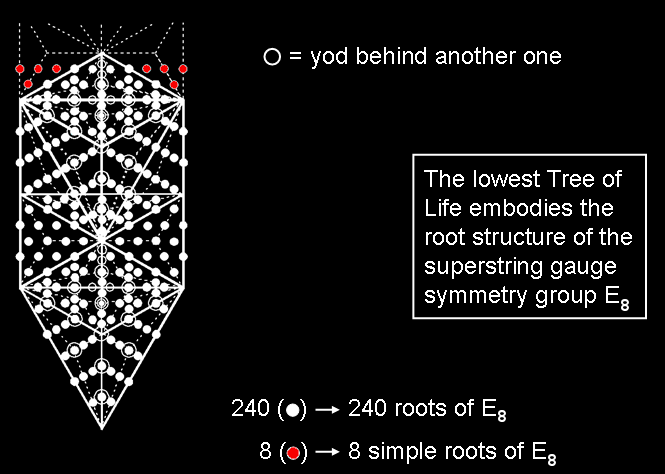The 1-tree is the lowest Tree of Life of any set of overlapping Trees of Life. When its 19 triangles are Type A, it contains 251 yods.* 11 of these are corners of these triangles to which the 10 Sephiroth and Daath are assigned. There are (251−11=240) white yods that are either hexagonal yods or internal corners of the the Type A triangles. Outside the 1-tree and below its apex are four red hexagonal yods on either side of the central Pillar of Equilibrium. Therefore, (240+8=248) yods lie below the top of the 1-tree that are not Sephirothic corners of triangles. The number 248 is both the gematria number value of Raziel, the Archangel of Chokmah, and the dimension of E8. The eight red yods outside the 1-tree symbolise the eight simple roots of E8 and the 240 yods that are not Sephirothic corners denote its 240 roots. See also #1 & #2

* Proof: The 19 triangles in the 1-tree have 25 sides and 11 corners. (25×2 + 11 = 61) yods line their sides. There are 10 yods inside each Type A triangle. Number of yods in the 1-tree = 61 + 19×10 = 251.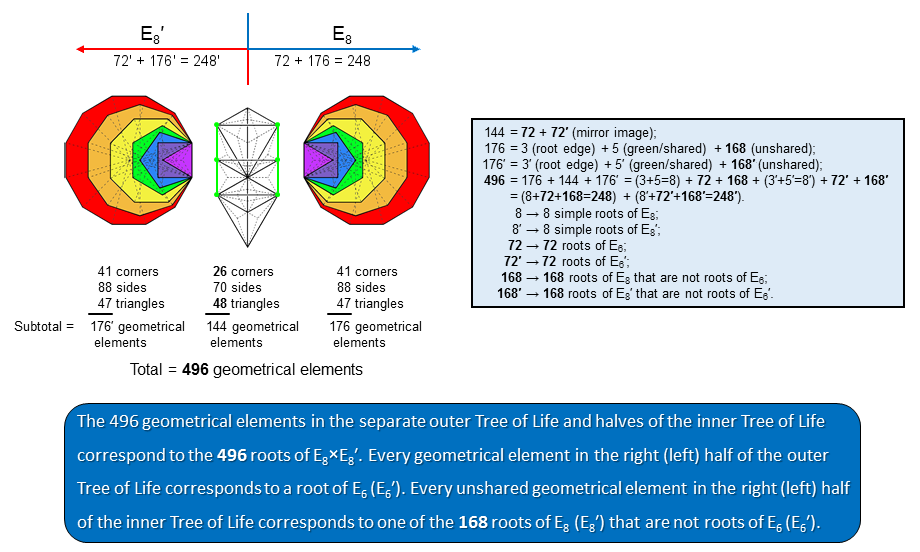Correspondence between the 496 roots of E8×E8 and the
geometrical composition of the separate outer & inner Trees of Life

The outer Tree of Life is composed of 16 triangles. When they are Type A, they have (16×3=48) sectors with (10+16=26) corners and (22 + 16×3 = 70) sides. These 144 geometrical elements comprise those distributed on either side of the central Pillar of Equilibrium as pairs of elements that are mirror images of each other and those either located on this Pillar or spanning both sides of it in a symmetrical way; the latter can be divided into two equal sets that can be formally associated with either half of the outer Tree of Life. This means that 72 geometrical elements are associated with each half if it. Those associated with the left-hand set of 7 polygons are written as 72′. The 7 enfolded polygons making up each half of the inner Tree of Life are composed of 47 triangles with 41 corners and 88 sides. These 176 geometrical elements comprise the three geometrical elements (two corners & one side) making up their shared root edge, the top, centre & bottom corner of the hexagon, the two vertical, internal sides of its sectors and 168 other geometrical elements. The hexagon in each set of polygons has 5 geometrical elements that coincide with their counterparts (coloured green in the diagram opposite) in the outer Tree when it combines with its inner form. The set of 5 shared elements in the left-hand set of polygons is written as 5′ and the 3 elements making up their root edge are written as 3′; their unshared elements outside the root edge are written as 168′. Hence,

144 = 72′ + 72,
176′ = 3′ + 5′ + 168′,
176 = 3 + 5 + 168.

The total number of geometrical elements in the separate outer and inner Trees with Type A polygons = 176′ + 144 + 176

= 496 = (3′+5′) + 72′ + 168′ + (3+5) + 72 + 168

= 8′ + 72′ + 168′ + 8 + 72 + 168 = 248′ + 248,

where 8′ ≡ 3′ + 5′ and 8 = 3 + 5. The 8 geometrical elements in the right-hand set of polygons that either belong to the root edge or are shared with the outer Tree of Life correspond to the 8 simple roots of E8; their 8 counterparts in the left-hand set (written as 8′) correspond to the 8 simple roots of an identical group (written as E8′). The 72 geometrical elements associated with either half of the outer Tree of Life correspond to the 72 roots of E6, an exceptional subgroup of E8. The remaining unshared 168 elements in each set of polygons correspond to the 168 roots of E8 that are not roots of E6. The direct product E8×E8 appearing in string theory as the symmetry group describing the unified interactions of one of the two types of heterotic superstrings arises from the left-right mirror symmetry of both the outer and inner forms of the Tree of Life. This natural isomorphism between the root composition of E8×E8 and the geometrical composition of the Tree of Life can have no other plausible explanation than that E8×E8 heterotic superstrings exist as the subatomic manifestation of the universal blueprint known as the "Tree of Life."

 << Previous    1...   50  51    53  54  ...56    Next >>

Home